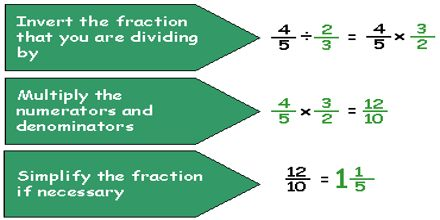Mathematic

# Dividing FractionsBasic purpose of this lecture is to present on Dividing Fractions. Here briefly describe how to calculate Dividing Fractions. Step 1. Invert the divisor. Step 2. Change the division sign to a multiplication sign. Step 3. Cross cancel if you can. Multiply the numerators. Multiply the denominators. To divide any number by a fraction: Multiply the number by the reciprocal of the fraction; Simplify the resulting fraction if possible. Check your answer: Multiply the result you got by the divisor and be sure it equals the original dividend.